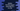# Python program to find the largest even and odd numbers in a list## Write a Python Program to print the largest Even and Odd numbers in a list :

In this tutorial, we will write one python 3 program to find out the largest odd and even number in a list.

The user will enter all the numbers to store in the list. Next, we will run one loop to find out the largest even and odd numbers. We will use one loop to read the numbers to the list. You can use one already populated list but we will take the numbers from the user as inputs here.

The list is a collection to hold items in python. All items are placed inside a square bracket []. The items are separated with a comma. A list can hold items of different datatypes. For this example, we are using only integer values.

To access an item in a list, the index is used. The index of the items starts from 0, i.e. the index of the first element is 0, the index of the second element is 1 etc. Using the index, we can access, modify or delete a list item.

The below algorithm we are going to use in this example :

### The algorithm used to find out the largest even and odd numbers :

1. Ask the user how many numbers he wants to add to the list.
2. Create one empty list to store all numbers.
3. Run one ‘for’ loop and get all the numbers from the user one by one. Read each number and add it to the list.
4. Create two variables to store the largest even and largest odd number of the list.
5. Now, run one for loop and scan all numbers of the user input list one by one.
6. Check for each number: if it is even and greater than the stored largest even number, assign it as the largest even. Do the same thing for the odd number also. The variables used to store the largest even number and the largest odd number will be updated continuously. At the end of the program, these variables will store the final largest even and odd numbers.
7. After all elements are scanned, print the largest odd and largest even number or print the variables used to hold the largest odd and even numbers.

### Python 3 Program :

``````# get the total numbers to store in the list
total_numbers = int(input("How my numbers you want to add to the list : "))

# create one empty array to store the numbers
numbers_array = []

# run a loop and get the inputs from the user
for i in range(0, total_numbers):
numbers_array.append(int(input("Number to add : ")))

# create two variables to store largest even and odd number of the list
# store -1 to both of these variables
largest_even = -1
largest_odd = -1

# Now scan the array again and update the largest value if found
for i in range(0, total_numbers):
if(numbers_array[i] % 2 == 0 and numbers_array[i] > largest_even):
# even number
largest_even = numbers_array[i]
elif(numbers_array[i] % 2 != 0 and numbers_array[i] > largest_odd):
# odd number
largest_odd = numbers_array[i]

# All numbers are scanned. Now print the largest odd and even value
print("Largest Odd Number : ", largest_odd)
print("Largest Even Number : ", largest_even)``````

You can also download the program from here.

### Example Output :

• In the above example, we have largest_odd and largest_even variables to hold the largest odd and even number of the list.
• In a similar way, we can also find out the smallest even and smallest odd numbers using python. For finding out the smallest even and odd numbers, we will have to define two numbers to hold the smallest numbers similar to the above program. We can scan the numbers in the list one by one and update these numbers if required.

### Conclusion :

In this tutorial, we have learned how to find the largest odd and even numbers in a list. In this program, we have initialized the largest odd and even numbers as -1. But we can also initialize both of them as the first number of the list and we can start the loop to iterate from the second number of the list.

Try to run the above example program and drop one comment below if you have any queries.